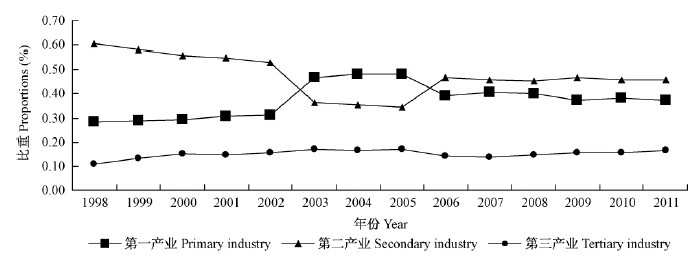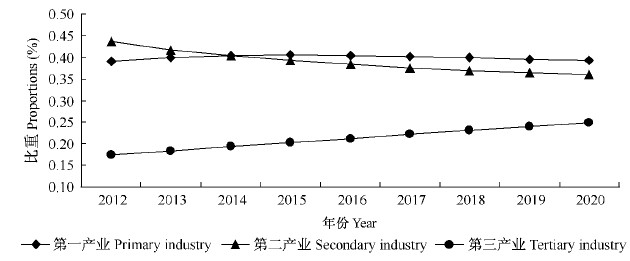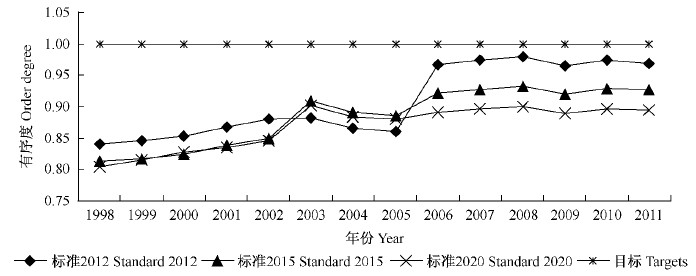﻿ 基于马尔科夫二次规划模型的黑龙江省林业产业结构的有序度测算与优化
 林业科学2014, Vol. 50Issue (9): 138-144PDF
DOI: 10.11707/j.1001-7488.20140919
0

#### 文章信息

Lü Jiehua, Zhang Hongrui, Zhang Bin

Order Degree Calculation and Optimization on Forestry Industrial Structure of Heilongjiang Province Based on Markov Quadratic Programming Model

Scientia Silvae Sinicae, 2014, 50(9): 138-144.
DOI: 10.11707/j.1001-7488.20140919

### 作者相关文章

Order Degree Calculation and Optimization on Forestry Industrial Structure of Heilongjiang Province Based on Markov Quadratic Programming Model
Lü Jiehua, Zhang Hongrui, Zhang Bin
College of Economics and Management,Northeast Forestry University Harbin 150040
Abstract: In this study, a quadratic programming model was used to obtain Markov transition matrix of the forestry industry structure, which was applied to predict the forestry target industrial structure of Heilongjiang province from 2012 to 2020. The calculated results of 2012, 2015 and 2020 were chosen as the reference standard, the development order degree of forestry industrial structure of Heilongjiang province from 1998 to 2011 was calculated, and the adjustment degree of forestry industrial structure of Heilongjiang province was quantitatively studied. The results showed that the forestry industry structure of Heilongjiang province was in process of dynamic optimization, especially in 2006-2011. The order degree values were successively among 0.97-0.98, 0.92-0.93 and 0.89-0.90,indicating that the adjustment degree of forestry industrial structure in Heilongjiang province in recent years was well, but the forestry industry still needs to be further adjusted. Based on the results and the actual situation of Heilongjiang forestry industry, the strategies to optimize the forestry industrial structure are put forward.
Key words: forestry industry structure    order degree    target industrial structure    Markov quadratic programming model    optimization strategies

1 产业结构有序度测算方法

Xi=[Xi(1)，Xi(2)，…，Xi(n)]为产业结构向量，令：

xi0(k)=Xi(k)-Xi(1)，k=1，2，…，n

Xi0=[xi0(1)，xi0(2)，…，xi0(n)]，为Xi的始点零化像。

X0=[x0(1)，x0(2)，…，x0(n)]为产业结构调整的目标向量，其始点零化像记作：

X00=[x00(1)，x00(2)，…，x00(n)]。

 \begin{align} & \left| {{s}_{0}} \right|=\sum\limits_{k=2}^{n-1}{\left| x_{0}^{0}\left(k \right)\right|+\frac{1}{2}}\left| x_{0}^{0}\left(n \right)\right|，\\ & \left| {{s}_{i}} \right|=\sum\limits_{k=2}^{n-1}{\left| x_{i}^{0}\left(k \right)\right|+\frac{1}{2}}\left| x_{i}^{0}\left(n \right)\right|，\\ & \left| {{s}_{i}}-{{s}_{0}} \right|=\sum\limits_{k=2}^{n-1}{\left| x_{i}^{0}\left(k \right)\right|-}\left| x_{0}^{0}\left(k \right)\right|+ \\ & \frac{1}{2}\left| x_{i}^{0}\left(n \right)-x_{0}^{0}\left(n \right)\right| \\ \end{align}

 ${{\varepsilon }_{0i}}=\frac{1+\left| {{s}_{0}} \right|+\left| {{s}_{i}} \right|}{1+\left| {{s}_{0}} \right|+\left| {{s}_{i}} \right|+\left| {{s}_{0}}-{{s}_{i}} \right|}$ (1)

2 目标产业结构方法的确定 2.1 目标产业结构的一般方法

2.2 马尔科夫趋势分析法

X(K+1)=X(KP

3 黑龙江省林业产业结构的目标标准测算 3.1 林业产业结构的马尔科夫二次规划模型

X(t)表示t时间黑龙江省林业产业总产值，Xi(t)为t时间林业第i产业的产值，根据转移关系有${{W}_{j}}\left(t \right)=\sum\limits_{i=1}^{3}{{{W}_{i}}\left(t-1 \right){{P}_{ij}}+{{\varepsilon }_{j}}\left(t \right)}$，只要求出使得$\sum\limits_{i=1}^{n-1}{\sum\limits_{j=1}^{3}{{{\left[ {{\varepsilon }_{j}}\left(t \right)\right]}^{2}}}}$达到最小的Pij，即得到黑龙江省林业产业结构的马尔科夫转移矩阵。

 $\min \sum\limits_{i=1}^{n-1}{\sum\limits_{j=1}^{3}{{{\left[ {{W}_{j}}\left( t \right)-\sum\limits_{i=1}^{3}{{{W}_{i}}\left( t-1 \right){{P}_{ij}}} \right]}^{2}}}}$ (2)

 \text{s}\text{.t}\text{.}\left\{ \begin{align} & \sum\limits_{i=1}^{3}{{{P}_{ij}}=1} \\ & {{P}_{ij}}\ge 0 \\ \end{align} \right.

3.2 基本假设

3.3 林业产业结构的马尔科夫二次规划结果图 1 1998—2011年黑龙江省林业产业结构 Fig. 1 Forestry industrial structure of Heilongjiang province between 1998 and 2011

 P=\left[ \begin{align} & 0.8368\text{ }0.1302\text{ }0.0330 \\ & 0.1669\text{ }0.8331\text{ }0 \\ & 0\text{ }0.0192\text{ }0.9808 \\ \end{align} \right]

 ${{A}_{\left(2011+k \right)}}=A\times {{P}^{k}}$ (3)图 2 2012—2020年黑龙江省林业3类产业预测值 Fig. 2 The predictive value line chart of three forestry industry of Heilongjiang Province from 2012 to 2020

4 黑龙江省林业产业结构的有序度测算结果图 3 1998—2011年黑龙江省林业产业结构有序度 Fig. 3 The order degree line chart of forestry industrial structure of Heilongjiang Province from 1998 to 2011
5 结论

6 产业整体优化思路与各产业优化策略 6.1 产业整体优化思路

6.2 各产业优化策略 6.2.1 吸纳更多剩余力量，巩固林业第一产业基础

6.2.2 淘汰落后次级产业，推动林业第二产业内部结构升级

6.2.3 发挥林业资源优势，增强林业第三产业发展潜力

  陈杰.2012.MATLAB宝典. 3版.北京:电子工业出社,190-191.(1)  曹颖,万志芳.2012.黑龙江省林业产业结构分析——基于偏离-份额分析法.林业经济,(11):102-104,115.(1)  党耀国,刘思峰,王庆丰.2011.区域产业结构优化理论与实践.北京:科学出版社,94-95,158-160.(1)  耿玉德,万志芳.2006.黑龙江省国有林区林业产业结构调整与优化研究.林业科学,42(6):86-93.(1)  姜玉砚.2013.产业结构有序度的测度、优化调整及预测——基于山西2001—2011年数据的分析.经济问题,(5):24-27.(1)  刘思峰,唐学文,袁潮清,等.2004.我国产业结构的有序度研究.经济学动态,(5):53-56.(1)  吕盈.2013.东北、内蒙古地区林业产业结构发展的灰色动态关联分析.福建农林大学学报: 哲学社会科学版,16(2):37-40.(1)  邵砾群,陈海滨,刘军弟.2012.基于灰色理论的陕西省林业产业结构分析预测.西北林学院学报,27(5):289-292.(1)  张波,商豪.2009.应用随机过程.北京:中国人民大学出版社,84-124.(1)  张帆.2012.江西省林业产业结构分析与优化研究.南京: 南京林业大学硕士学位论文. (1)  Clark C.1967.The conditions of economic progress. Madrid: Alianza Editorial.(1)  Leontief W.1953.Domestic production and foreign trade; the American capital position re-examined. Proceedings of the American Philosophical Society,97(4): 332-349.(1)  Morris B.2001.Can differences in industry structure explain divergences in regional economic growth. Bank of England Quarterly Bulletin,41(2):195-203.(1)  Metcalfe S C.2006.Communal land reform in ZAMBIA: governance, livelihood and conservation. Bellville, Sonth Africa:University of the Western Cape.(1)  Peneder M.2003.Industry structure and aggregate growth. Structure Change & Economic Dynamics,14(12):427-449.(1)  Zhang J, Jefferson G H.2009.Structural reforms and China's industrial growth. Economic Research,(7):4-20.(1)